## Seaborn 数据可视化基础教程

3411 字 · 9374 阅读 · 2019 年 06 月 01 日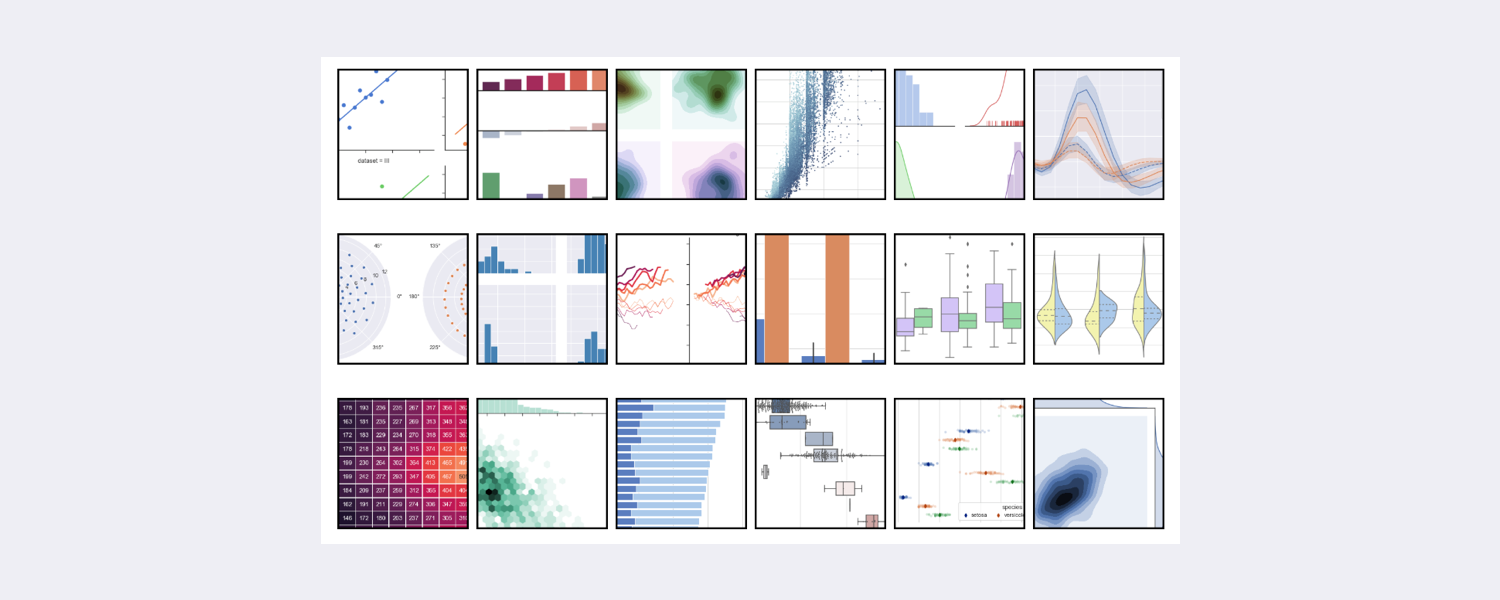Matplotlib 应该是基于 Python 语言最优秀的绘图库了，但是它也有一个十分令人头疼的问题，那就是太过于复杂了。3000 多页的官方文档，上千个方法以及数万个参数，属于典型的你可以用它做任何事，但又无从下手。尤其是，当你想通过 Matplotlib 调出非常漂亮的效果时，往往会伤透脑筋，非常麻烦。

Seaborn 基于 Matplotlib 核心库进行了更高阶的 API 封装，可以让你轻松地画出更漂亮的图形。Seaborn 的漂亮主要体现在配色更加舒服、以及图形元素的样式更加细腻，下面是 Seaborn 官方给出的参考图。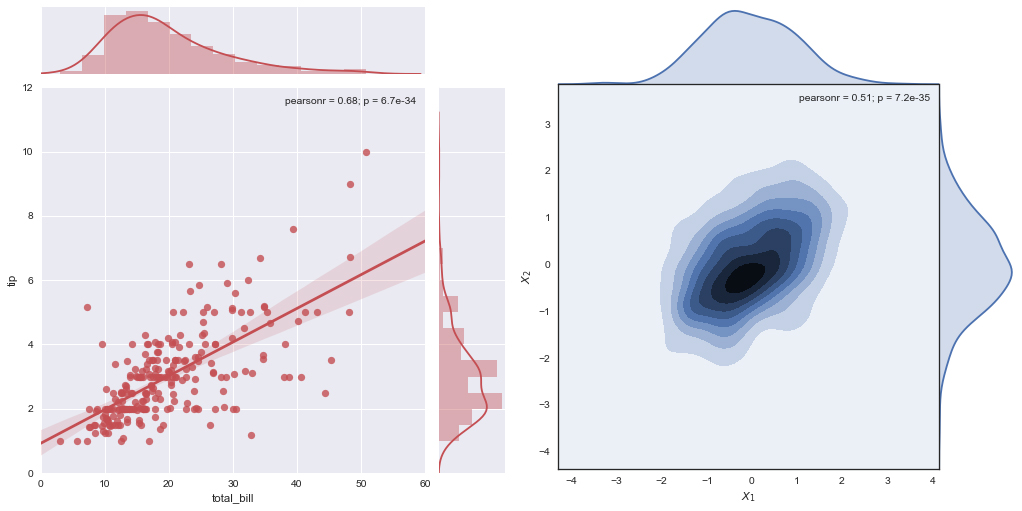Seaborn 具有如下特点：

• 内置数个经过优化的样式效果。
• 增加调色板工具，可以很方便地为数据搭配颜色。
• 单变量和双变量分布绘图更为简单，可用于对数据子集相互比较。
• 对独立变量和相关变量进行回归拟合和可视化更加便捷。
• 对数据矩阵进行可视化，并使用聚类算法进行分析。
• 基于时间序列的绘制和统计功能，更加灵活的不确定度估计。
• 基于网格绘制出更加复杂的图像集合。

### 快速优化图形

import matplotlib.pyplot as plt
%matplotlib inline

x = [1, 3, 5, 7, 9, 11, 13, 15, 17, 19]
y_bar = [3, 4, 6, 8, 9, 10, 9, 11, 7, 8]
y_line = [2, 3, 5, 7, 8, 9, 8, 10, 6, 7]

plt.bar(x, y_bar)
plt.plot(x, y_line, '-o', color='y')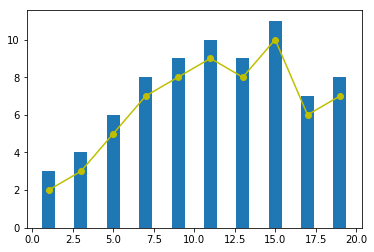import seaborn as sns

sns.set()  # 声明使用 Seaborn 样式

plt.bar(x, y_bar)
plt.plot(x, y_line, '-o', color='y')sns.set() 的默认参数为：

sns.set(context='notebook', style='darkgrid', palette='deep', font='sans-serif', font_scale=1, color_codes=False, rc=None)


• context='' 参数控制着默认的画幅大小，分别有 {paper, notebook, talk, poster} 四个值。其中，poster > talk > notebook > paper
• style='' 参数控制默认样式，分别有 {darkgrid, whitegrid, dark, white, ticks}，你可以自行更改查看它们之间的不同。
• palette='' 参数为预设的调色板。分别有 {deep, muted, bright, pastel, dark, colorblind} 等，你可以自行更改查看它们之间的不同。
• 剩下的 font='' 用于设置字体，font_scale= 设置字体大小，color_codes= 不使用调色板而采用先前的 'r' 等色彩缩写。

### Seaborn 绘图 API

Seaborn 一共拥有 50 多个 API 类，相比于 Matplotlib 数千个的规模，可以算作是短小精悍了。其中，根据图形的适应场景，Seaborn 的绘图方法大致分类 6 类，分别是：关联图、类别图、分布图、回归图、矩阵图和组合图。而这 6 大类下面又包含不同数量的绘图函数。

#### 关联图

relplot 绘制关系图
scatterplot 多维度分析散点图
lineplot 多维度分析线形图

relplot 是 relational plots 的缩写，其可以用于呈现数据之后的关系，主要有散点图和条形图 2 种样式。我们载入鸢尾花示例数据集。

iris = sns.load_dataset("iris")

sepal_length sepal_width petal_length petal_width species
0 5.1 3.5 1.4 0.2 setosa
1 4.9 3.0 1.4 0.2 setosa
2 4.7 3.2 1.3 0.2 setosa
3 4.6 3.1 1.5 0.2 setosa
4 5.0 3.6 1.4 0.2 setosa

sns.relplot(x="sepal_length", y="sepal_width", data=iris)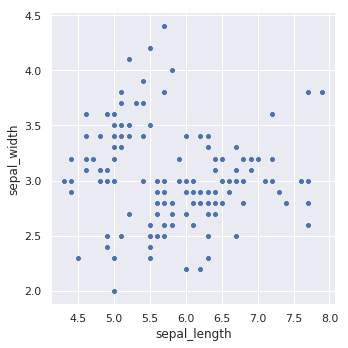sns.relplot(x="sepal_length", y="sepal_width", hue="species", data=iris)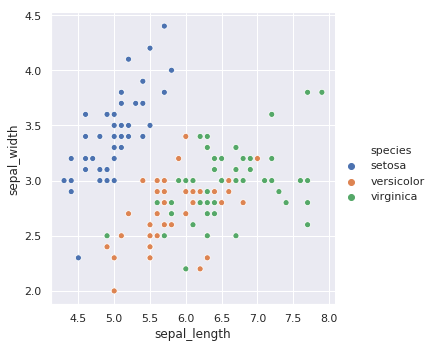Seaborn 的函数都有大量实用的参数，例如我们指定 style 参数可以赋予不同类别的散点不同的形状。更多的参数，希望大家通过阅读官方文档了解。

sns.relplot(x="sepal_length", y="sepal_width",
hue="species", style="species", data=iris)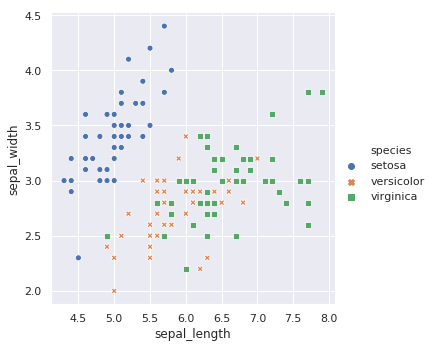sns.relplot(x="sepal_length", y="petal_length",
hue="species", style="species", kind="line", data=iris)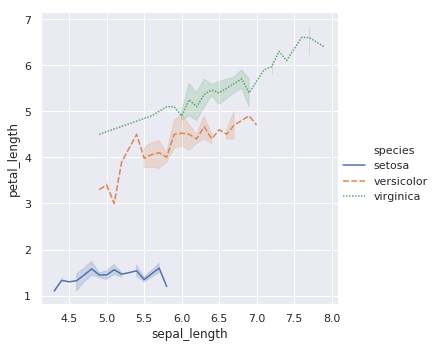Figure-level 和 Axes-level API 的区别在于，Axes-level 的函数可以实现与 Matplotlib 更灵活和紧密的结合，而 Figure-level 则更像是「懒人函数」，适合于快速应用。

sns.lineplot(x="sepal_length", y="petal_length",
hue="species", style="species", data=iris)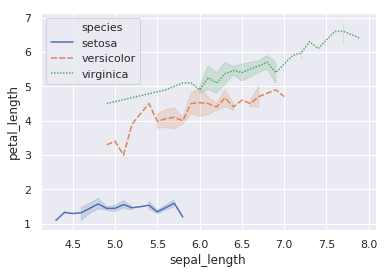#### 类别图

sns.catplot(x="sepal_length", y="species", data=iris)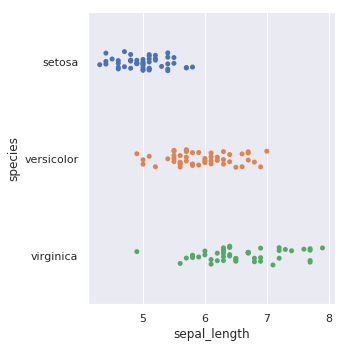kind="swarm" 可以让散点按照 beeswarm 的方式防止重叠，可以更好地观测数据分布。

sns.catplot(x="sepal_length", y="species", kind="swarm", data=iris)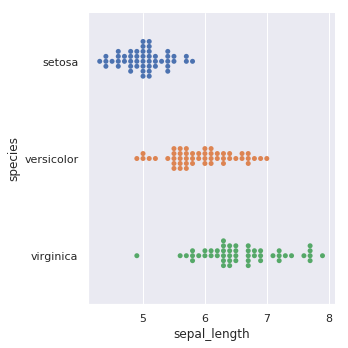sns.catplot(x="sepal_length", y="species", kind="box", data=iris)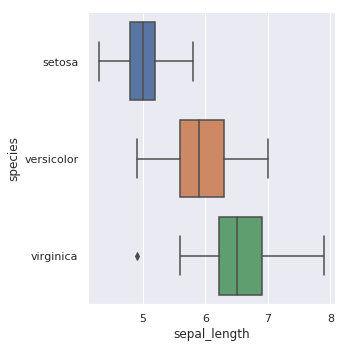sns.catplot(x="sepal_length", y="species", kind="violin", data=iris)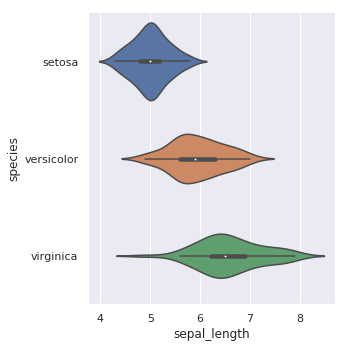sns.catplot(x="species", y="sepal_length", kind="boxen", data=iris)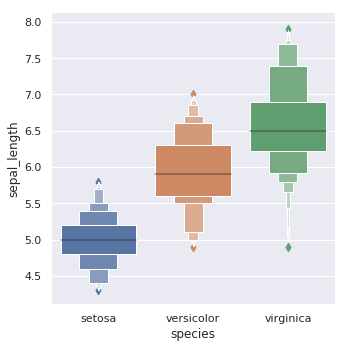sns.catplot(x="sepal_length", y="species", kind="point", data=iris)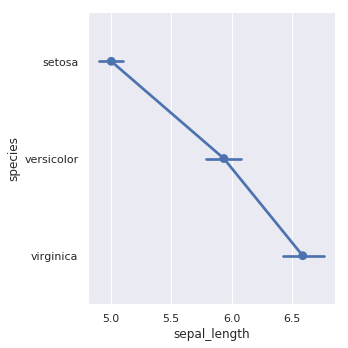sns.catplot(x="sepal_length", y="species", kind="bar", data=iris)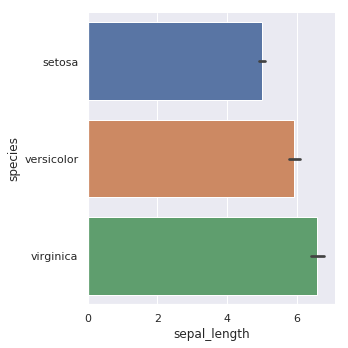sns.catplot(x="species", kind="count", data=iris)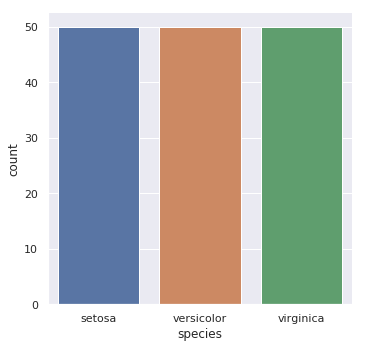#### 分布图

Seaborn 提供的分布图绘制方法一般有这几个：
jointplotpairplotdistplotkdeplot。接下来，我们依次来看一下这些绘图方法的使用。

Seaborn 快速查看单变量分布的方法是 distplot。默认情况下，该方法将会绘制直方图并拟合核密度估计图。

sns.distplot(iris["sepal_length"])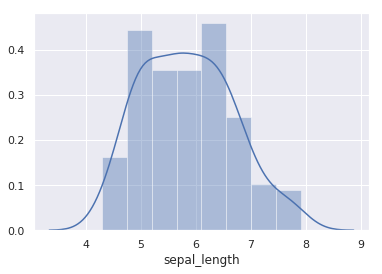distplot 提供了参数来调整直方图和核密度估计图，例如设置 kde=False 则可以只绘制直方图，或者 hist=False 只绘制核密度估计图。当然，kdeplot 可以专门用于绘制核密度估计图，其效果和 distplot(hist=False) 一致，但 kdeplot 拥有更多的自定义设置。

sns.kdeplot(iris["sepal_length"])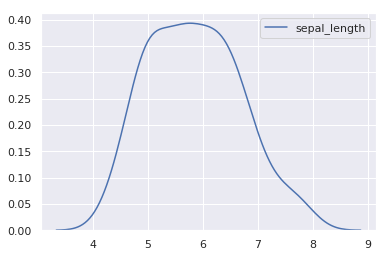jointplot 主要是用于绘制二元变量分布图。例如，我们探寻 sepal_lengthsepal_width 二元特征变量之间的关系。

sns.jointplot(x="sepal_length", y="sepal_width", data=iris)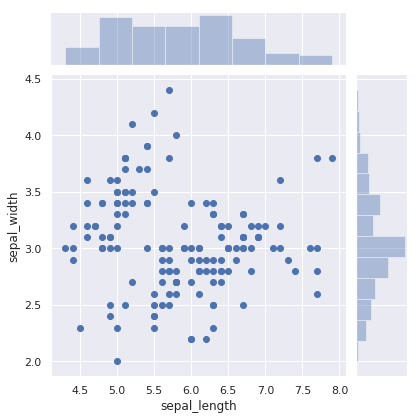jointplot 并不是一个 Figure-level 接口，但其支持 kind= 参数指定绘制出不同样式的分布图。例如，绘制出核密度估计对比图。

sns.jointplot(x="sepal_length", y="sepal_width", data=iris, kind="kde")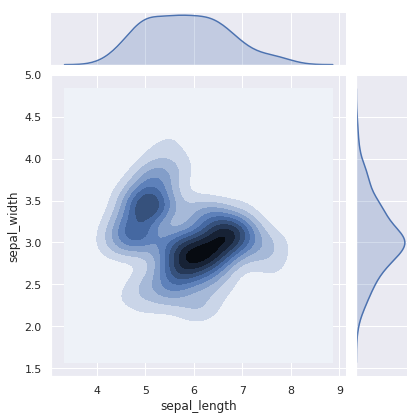sns.jointplot(x="sepal_length", y="sepal_width", data=iris, kind="hex")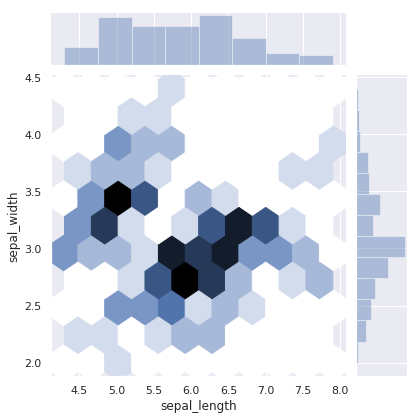sns.jointplot(x="sepal_length", y="sepal_width", data=iris, kind="reg")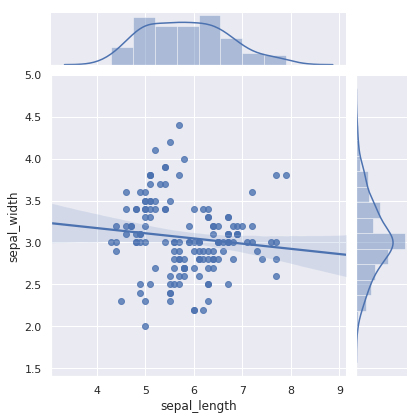sns.pairplot(iris)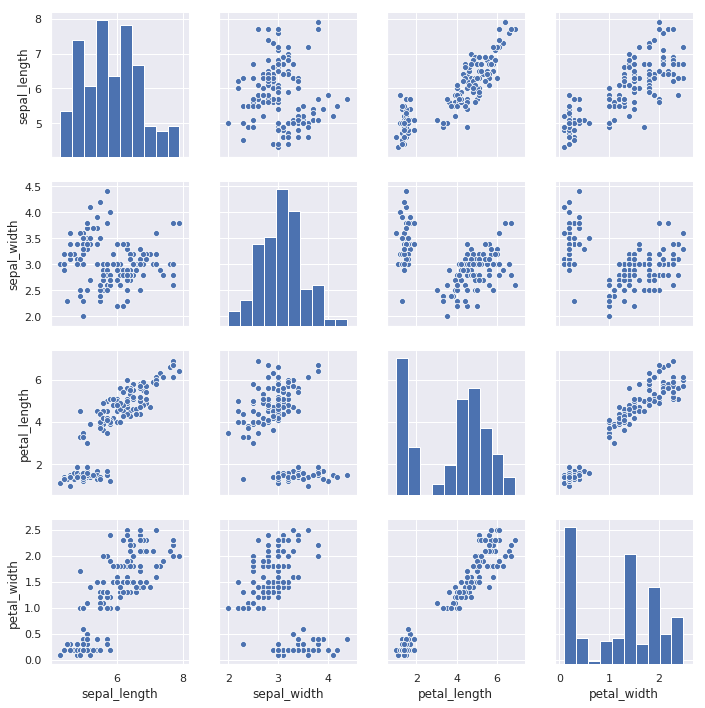sns.pairplot(iris, hue="species")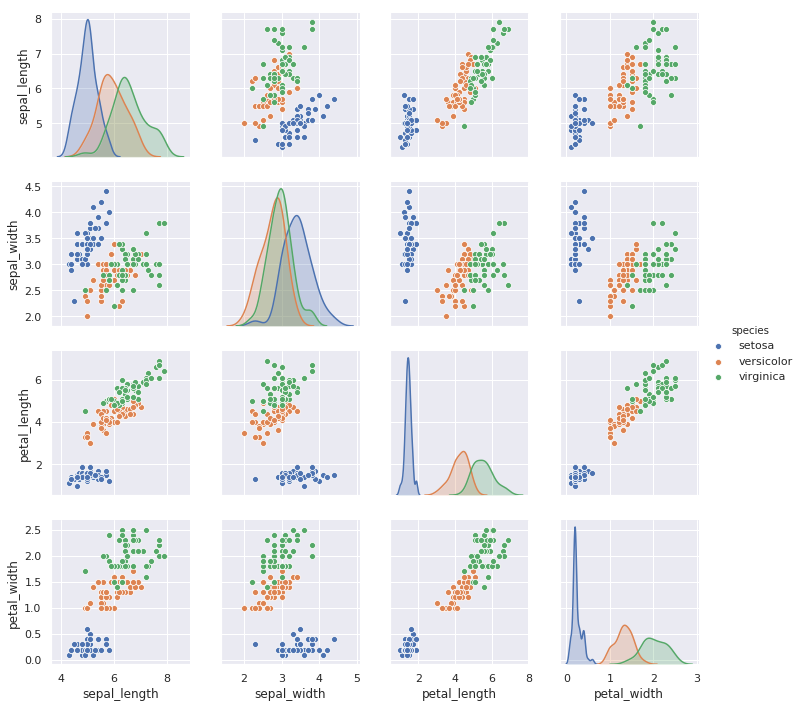#### 回归图

regplot 绘制回归图时，只需要指定自变量和因变量即可，regplot 会自动完成线性回归拟合。

sns.regplot(x="sepal_length", y="sepal_width", data=iris)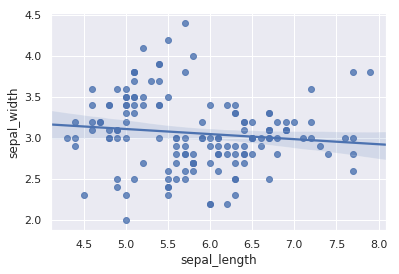lmplot 同样是用于绘制回归图，但 lmplot 支持引入第三维度进行对比，例如我们设置 hue="species"

sns.lmplot(x="sepal_length", y="sepal_width", hue="species", data=iris)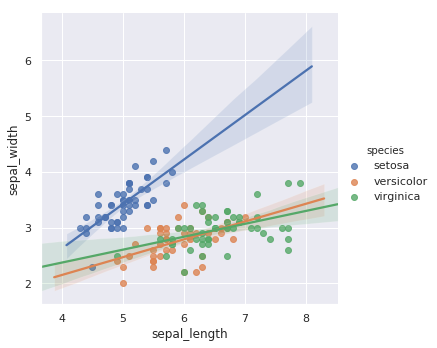#### 矩阵图

import numpy as np

sns.heatmap(np.random.rand(10, 10))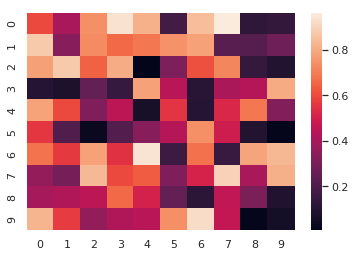iris.pop("species")
sns.clustermap(iris)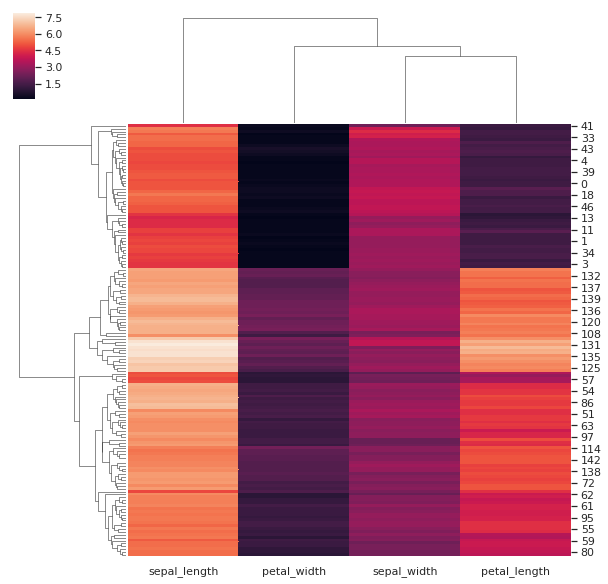#### 在线学习•